Search by Topic

Filter by: Content type:
Age range:
Challenge level:

There are 23 NRICH Mathematical resources connected to Pinboard/geoboard, you may find related items under Mathematics Tools.

Broad Topics > Mathematics Tools > Pinboard/geoboard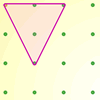Inside Triangles

Age 5 to 7 Challenge Level:

How many different triangles can you draw on the dotty grid which each have one dot in the middle?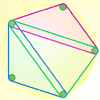Board Block Challenge

Age 7 to 11 Challenge Level:

Choose the size of your pegboard and the shapes you can make. Can you work out the strategies needed to block your opponent?Nine-pin Triangles

Age 7 to 11 Challenge Level:

How many different triangles can you make on a circular pegboard that has nine pegs?Right Angles

Age 11 to 14 Challenge Level:

Can you make a right-angled triangle on this peg-board by joining up three points round the edge?Subtended Angles

Age 11 to 14 Challenge Level:

What is the relationship between the angle at the centre and the angles at the circumference, for angles which stand on the same arc? Can you prove it?Triangles in Circles

Age 11 to 14 Challenge Level:

Can you find triangles on a 9-point circle? Can you work out their angles?Pick's Theorem

Age 14 to 16 Challenge Level:

Polygons drawn on square dotty paper have dots on their perimeter (p) and often internal (i) ones as well. Find a relationship between p, i and the area of the polygons.Transformations on a Pegboard

Age 7 to 11 Challenge Level:

How would you move the bands on the pegboard to alter these shapes?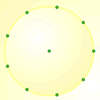Age 7 to 11 Challenge Level:

How many DIFFERENT quadrilaterals can be made by joining the dots on the 8-point circle?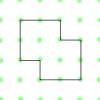Happy Halving

Age 5 to 7 Challenge Level:

Can you split each of the shapes below in half so that the two parts are exactly the same?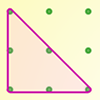Take a ... Geoboard

Age 5 to 11

This article for teachers explains why geoboards are such an invaluable resource and introduces several tasks which make use of them.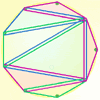Board Block for Two

Age 5 to 7 Challenge Level:

Board Block game for two. Can you stop your partner from being able to make a shape on the board?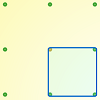Peg and Pin Boards

Age 5 to 11

This article for teachers suggests activities based on pegboards, from pattern generation to finding all possible triangles, for example.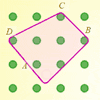Elastic Maths

Age 14 to 18

How do you write a computer program that creates the illusion of stretching elastic bands between pegs of a Geoboard? The answer contains some surprising mathematics.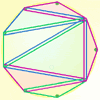Board Block

Age 5 to 7 Challenge Level:

Take it in turns to make a triangle on the pegboard. Can you block your opponent?Triangle Pin-down

Age 7 to 11 Challenge Level:

Use the interactivity to investigate what kinds of triangles can be drawn on peg boards with different numbers of pegs.Triangles All Around

Age 7 to 11 Challenge Level:

Can you find all the different triangles on these peg boards, and find their angles?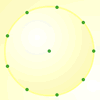Round Peg Board

Age 5 to 11 Challenge Level:

A generic circular pegboard resource.Dotty Triangles

Age 11 to 14 Challenge Level:

Imagine an infinitely large sheet of square dotty paper on which you can draw triangles of any size you wish (providing each vertex is on a dot). What areas is it/is it not possible to draw?Pinned Squares

Age 14 to 16 Challenge Level:

What is the total number of squares that can be made on a 5 by 5 geoboard?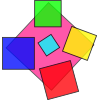Making Squares

Age 7 to 11

Investigate all the different squares you can make on this 5 by 5 grid by making your starting side go from the bottom left hand point. Can you find out the areas of all these squares?Tri.'s

Age 7 to 11 Challenge Level:

How many triangles can you make on the 3 by 3 pegboard?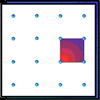Geoboards

Age 7 to 11 Challenge Level:

This practical challenge invites you to investigate the different squares you can make on a square geoboard or pegboard.